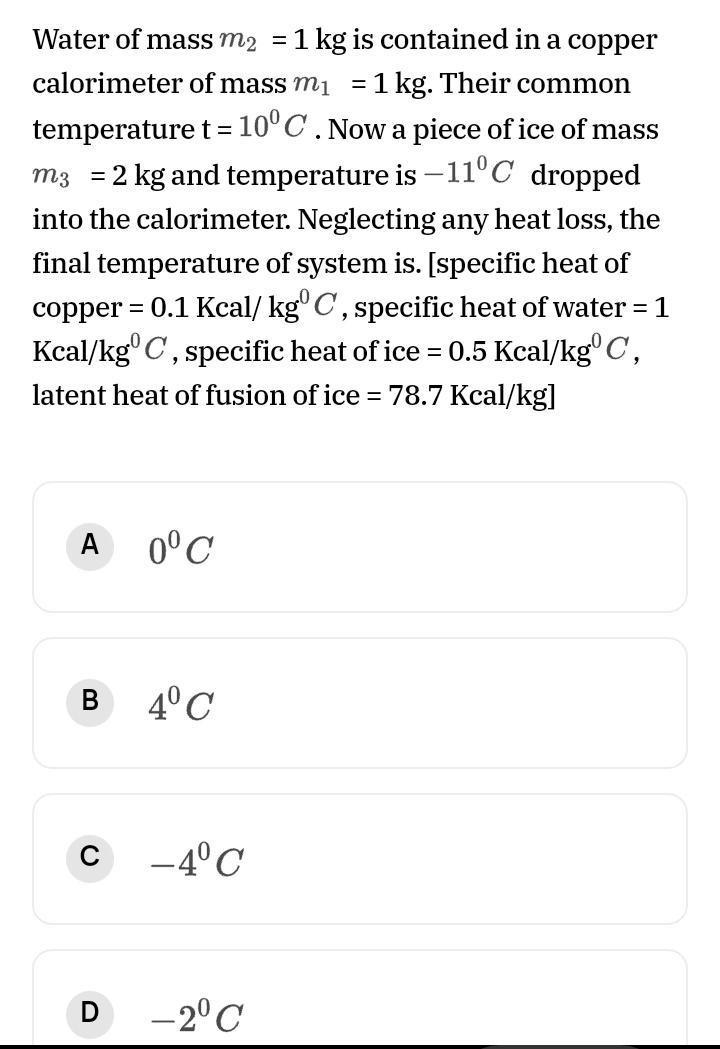Question:

# Water of mass m2 = 1 kg is contained in a copper calorimeter of mass mi = 1 kg. Their common temperature t = 10°C .Now a piece oWater of mass m2 = 1 kg is contained in a copper calorimeter of mass mi = 1 kg. Their common temperature t = 10°C .Now a piece of ice of mass m3 = 2 kg and temperature is -11°C dropped into the calorimeter. Neglecting any heat loss, the final temperature of system is. [specific heat of copper = 0.1 Kcal/ kg° C, specific heat of water = 1 Kcal/kg°C, specific heat of ice = 0.5 Kcal/kg°C, latent heat of fusion of ice = 78.7 Kcal/kg] A 0°C B 4°C C -4°C D -2°C